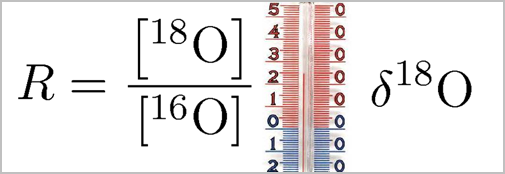# Isotopes and the delta notation

All water molecules consist of two Hydrogen atoms and one Oxygen atom, but there are different stable isotopes of Hydrogen and Oxygen.

The table shows the approximate occurrence rates of naturally occurring Hydrogen and Oxygen:

 1H 2H 3H (radioactive) 99.985% 0.015% varies

 16O 17O 18O 99.759% 0.037% 0.204%

The vast majority of water molecules consist of two 1H and one 16O atom. The two forms next in line are the forms with a heavy 18O isotope, denoted H218O, or with one ordinary Hydrogen atom replaced by a Deuterium atom, denoted HD16O, using the convention that 2H often is called D for Deuterium. Both H218O and HD16O molecules are heavier than the standard H216O molecule.

Due to their increased weight, the heavier water molecules evaporate slower from a water surface and form precipitation faster from a cloud than H216O.

The snow that precipitates on the Greenland ice sheet is therefore depleted in the heavier water molecules compared to ocean water. There is only about 2‰ H218O in natural waters, and in Arctic precipitation the amount of H218O is lowered by about 40‰ of the 2‰. The differences between the H218O content in precipitation are therefore very small, and a special unit has been invented to describe the amount of H218O in samples.

First, one measures (or calculates) the ratio of the molecules with heavy isotopes relative to the standard molecules:

 R = amount of H218O amount of H216O
or
 R = amount of HD16O amount of H216O

Next step is to calculate the relative deviation of R for a measured sample from the R of a standard:

 δ18O = Rsample−Rstandard ·1000‰ Rstandard
or
 δD = Rsample − Rstandard ·1000‰ Rstandard

The result is always given in permille.

Any standard can be used, but the most commonly used is "Standard Mean Ocean Water" (SMOW) or Vienna SMOW (VSMOW).